# Rounding Decimals to the Nearest Whole Number

Rounding Decimals to the Nearest Whole Number• Rounding a decimal to the nearest whole number means to write down the whole number that the decimal number is closest to.
• The decimal number of 1.3 is in between the whole numbers of 1 and 2.
• 1.5 is directly in between 1 and 2.
• 1.3 is closer to 1 than it is to 2 because it is on the left of 1.5.
• We look at the first decimal place, immediately after the decimal point to decide whether to round up or down to the nearest whole number.• To decide whether to round up or down, look at the first decimal place, immediately after the decimal point.
• If this digit is less than 5 then round down.
• Rounding down to the nearest whole number has the same effect as removing the decimal point and everything after it.
• 1.3 rounds down because the ‘3’ is less than ‘5’ and it is rounded down to 1.
• If the digit immediately after the decimal point is 5 or more, then round up.

Look at the digit immediately after the decimal point.

If the digit is 5 or more, round up.

Otherwise, if the digit is 4 or less, round down.• We will round the decimal number 17.491 to the nearest whole number.
• The choice of two numbers to round to will be the part of the number before the decimal point or the next number after this.
• The part of 17.491 before the decimal point is ’17’ and the number after 17 is ’18’.
• 17.491 can round down to 17 or round up to 18.
• To decide whether to round up or down look at the first digit after the decimal point.
• The rule is if the first decimal place is 5 or more, round up, otherwise round down.
• The digit after the decimal point is a ‘4’, which is less than 5 and so, we round down.
• 17.491 rounds down to 17 because it is nearer to 17 than 18.
• We can see on the number line that 17.491 is on the left of 17.5, which is mid-way between 17 and 18.Supporting Lessons#### Rounding Decimals to the Nearest Whole Number: Interactive Questions

Rounding Decimals to the Nearest Whole Number: Interactive Questions

# How to Round a Decimal Number to the Nearest Whole Number

To round a decimal to the nearest whole number we follow these steps:

• Look at the digit immediately after the decimal point.
• If this digit is 5 or more, then round up.
• Instead, if this digit is 4 or less, then round down.
• Rounding a decimal to the nearest whole number means to write down the whole number that the decimal number is closest to in size.

Rounding down to the nearest whole number means to write down the whole number which is immediately before the decimal number.

Rounding up to the nearest whole number means to write down the whole number that is immediately after the decimal number.

In the example below, we are asked to round the decimal number of 1.3 to the nearest whole number.We want to know which whole number is closest to 1.3.

We can see from the number line, that 1.3 is in between 1 and 2.

The whole number before the decimal point in ‘1.3’ is ‘1’.

We need to choose whether we round down to ‘1’ or round up to the next number, ‘2’.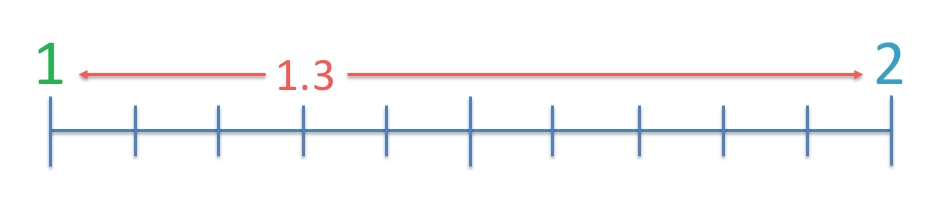We can see on the number line, that 1.3 is nearer to ‘1’ than it is to ‘2’.

We can see that 1.5 is directly in between 1 and 2.

The first decimal place is the digit immediately after the after the decimal point. In the number of 1.3, the first decimal place is ‘3’, since ‘3’ appears immediately after the decimal point.

Because ‘3’ is less than 5, we know that we need to round down because 1.3 is nearer to 1 than 2.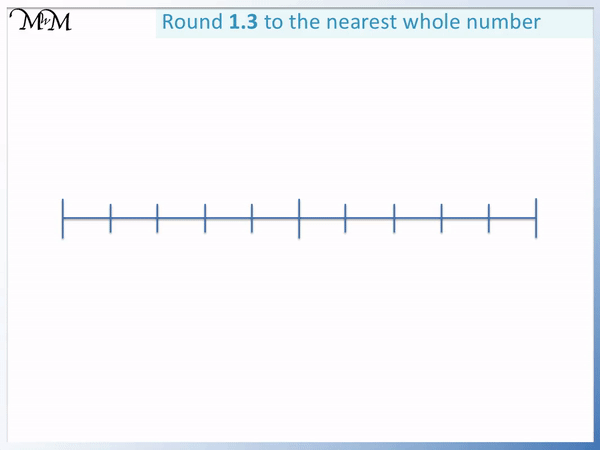In the next example we look at rounding the decimal number 1.6 to the nearest whole number.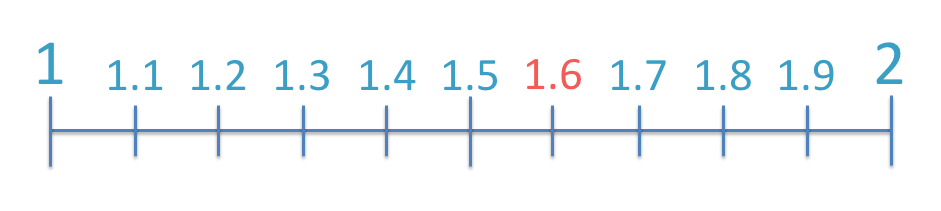Again the number 1.6 will round down to ‘1’ or round up to ‘2’.

We know this because the choice is to round to the part of the number directly before the decimal point, or the number after this.

The digit before the decimal point of 1.6 is ‘1’ and the number after 1 is 2.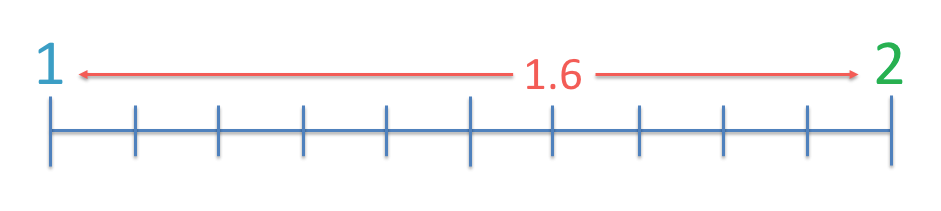We can see that 1.6 is nearer to 2 than to 1.

We round 1.6 up to 2.

Again we can use our rule to do this.

We look at the digit after the decimal point. This digit is ‘6’.

6 is ‘5 or more’ and so we round up.In the example below we are asked to round 1.5 to the nearest whole number.

We can see that 1.5 is actually directly in between the whole numbers of 1 and 2.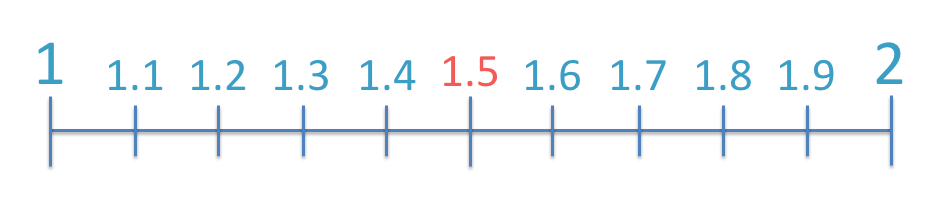We need to decide whether to round 1.5 up to 2 or down to 1.We use our rule to decide whether to round up or down.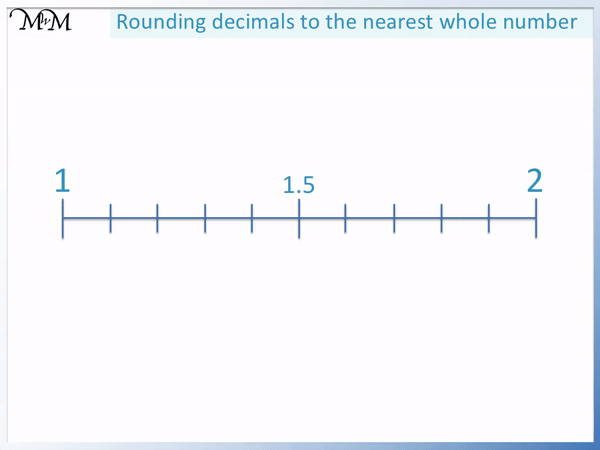If the digit after the decimal point is 5 or more, round up.

If the digit after the decimal point is 4 or less, round down.

The digit is ‘5’ and so it is included in the rule to round up.We round 1.5 up to 2.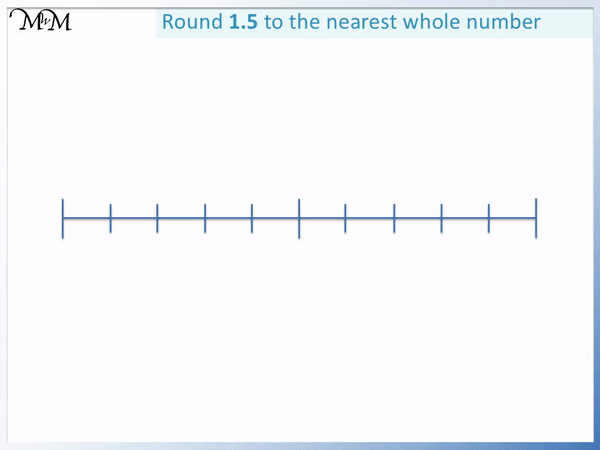The reason we choose to round the digit 5 up rather than down is because if there are any further digits after the ‘5’ then we will be nearer to the number above.

For example, all of the numbers: 1.51, 1.58, 1.50001 etc. are slightly nearer to 2 than they are to 1.

1.5 is directly in the middle of 1 and 2, however if there are any digits at all after the 5 then we move to be nearer to the number above.

If we include 5 in our rule to round up, then the rule will still work for any numbers that contain a 5 in the first decimal place.

When teaching and introducing rounding it can be helpful to begin by showing the size of the decimal numbers on a number line. However once the method is understood, it is better to move on to looking at the first decimal place to decide.

We will now look at some examples of rounding decimals to whole numbers by just using this rule, rather than drawing them on a number line.

In this example we are asked to round 2.8 to the nearest whole number.

The choice is to round down to 2 or round up to 3.

We look at the first decimal place, which is ‘8’.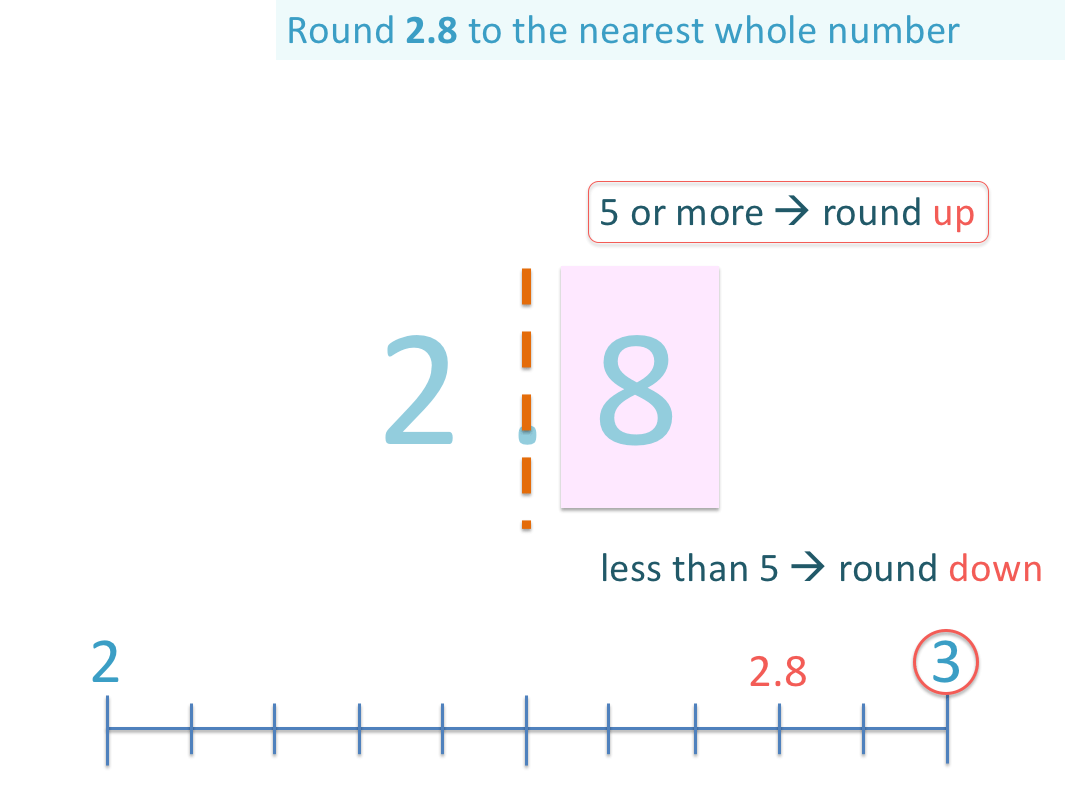If the digit after the decimal point is 5 or more, we round up.

The digit of ‘8’ is 5 or more and so we round up.

2.8 is nearer to 3 than it is to 2.

2.8 rounds up to 3 when written to the nearest whole number.Next we look at the decimal number of 5.38.

Only look at the digit that is immediately after the decimal point.

This is a ‘3’.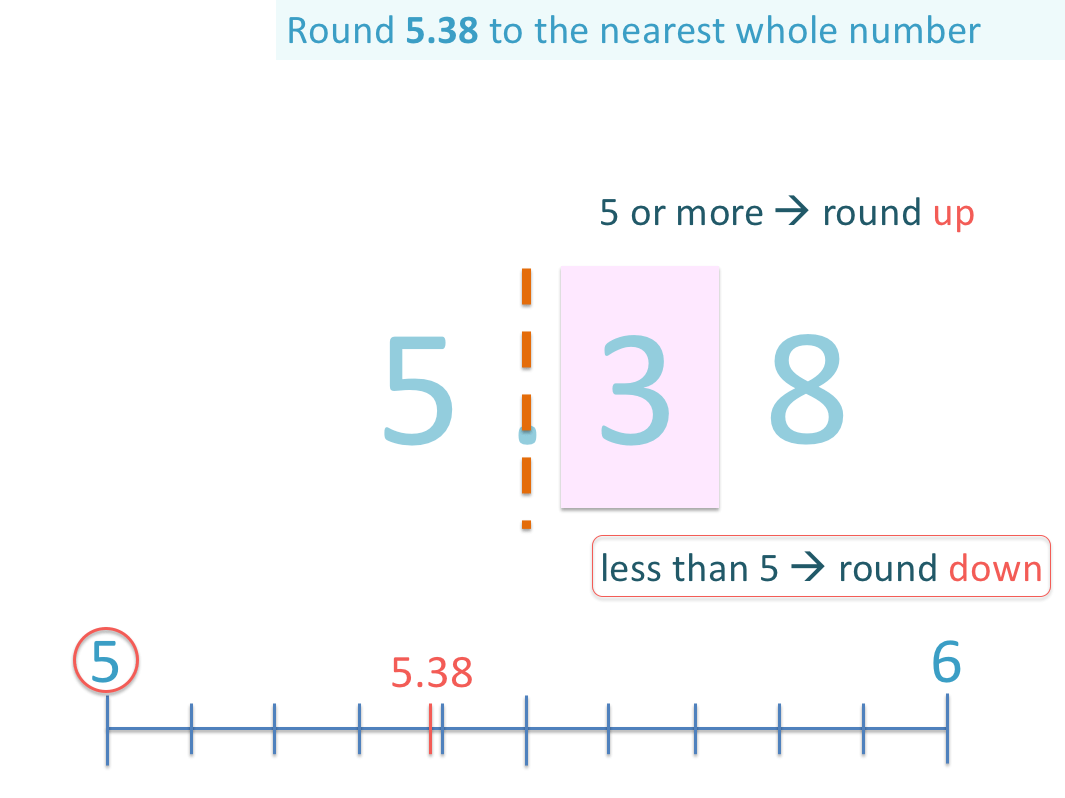The rule for rounding this number is to decide whether the digit in the first decimal place is 5 or more or 4 or less.

The digit ‘3’ is 4 or less and so, we round down 5.38 to 5.

Notice that we did not use the digit ‘8’ at the end of the decimal to help us decide. Do not look at any digits past the first decimal place to decide whether to round up or down.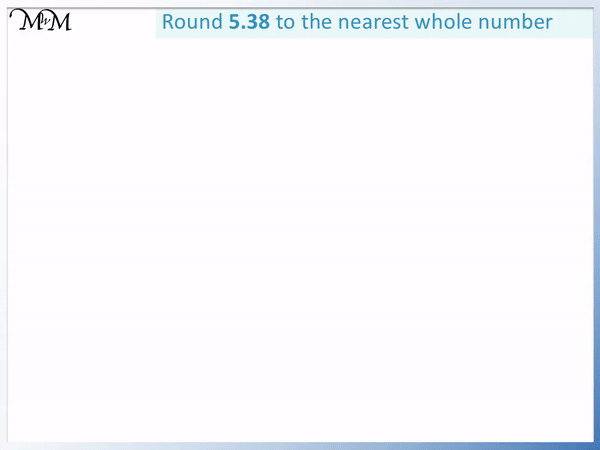Here is another example with 3 decimal places.

We are asked to round 17.491 to the nearest whole number. We choose between rounding down to 17 or rounding up to 18.

The digit after the decimal point is ‘4’.

And so we round down to 17.A common mistake is to look past the first decimal place at the ‘9’. It is common to look at the 9 and use it to round the 4 up to a 5, which then rounds the whole number up to 18 because it is a 5.

We do not do this. We only look at the digit immediately after the decimal point and use this digit alone to decide.

When rounding numbers, we never round up multiple times in the same number like in the common mistake mentioned above.

This is such a common mistake that when teaching rounding, it is worth point this out.

To overcome this mistake, it helps to show the size of the number on a number line.

17.491 is nearer to 17 than it is to 18 because it is on the left of 17.5, which is halfway.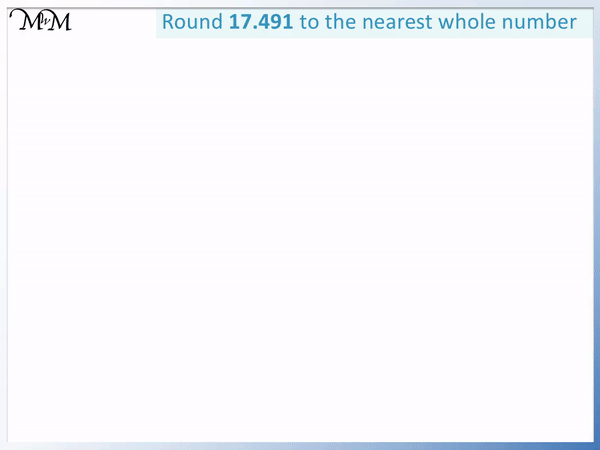The next example of rounding a decimal to the nearest whole number is 29.503.

We look at the digit immediately after the decimal point. If it is 5 or more, we round up. If it is 4 or less, we round down.

The digit after the decimal point is a ‘5’, and so, we round up.We can see that the number 29.503 is slightly nearer to 30 than it is to 29.

29.503 rounds up to 30, when written to the nearest whole number.Don’t forget to use our online question generator for infinite practise of rounding decimals to whole numbers.Now try our lesson on Converting Decimals to Percentages where we learn how to write a decimal as a percentage.